Manual calculation mode debuted in VisiCalc in 1979 and hasn’t been changed in 44 years. When you change the calculation mode to Manual, Excel will stop calculating. This can be useful to allow you to perform data entry without having a long delay to calculate cells after each entry.

When you’re in Manual calculation mode, Excel keeps track of which cells are out of date and need to be recalculated. These cells are called “stale” by Microsoft. With the new feature, you can choose to format stale values. Excel will add strikethrough to all cells that need to be recalculated.

There are two places to choose the calculation mode. You can either use the Calculation Options drop-down menu on the Formulas tab (Figure 1) or select File, Options, Formulas. In Figure 1, the calculation mode is changed to Manual and the new Format Stale Values option is enabled.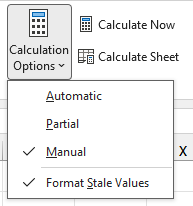Note: The calculation mode formerly called “Automatic Except Tables” is being renamed to “Partial.” This mode is used to pause calculation in Data Tables created using What-If tools. The Python programming language is being added to Excel. Microsoft needed a method to pause Python calculations so it changed the meaning of “Automatic Except Tables” to “Automatic Except Tables and Python.” But this was deemed too wordy, so the option is now called Partial.

Caution: Calculation mode is a global setting. If you have several workbooks open and change one workbook from Automatic to Manual, that setting will be applied to all open workbooks.

To illustrate the Format Stale Values feature, I’ve built a simple forecasting worksheet as shown in Figure 2. Enter predicted sales in the January column, and the model will calculate future sales by adding 10% each month. A Total row, Running Total row, and Total column are included to help illustrate which values are stale.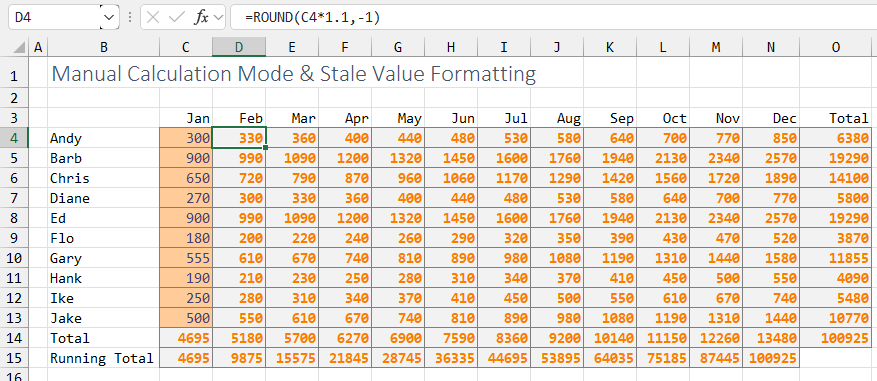In Figure 3, I’ve changed the January sales for Diane, Ed, and Flo to 500. Excel applies strikethrough to D7:O9 and also to the Totals and Running Totals in rows 14 and 15.

Note: I’m a frequent user of the Ctrl+5 shortcut to apply strikethrough to a cell. As someone who frequently uses strikethrough, I don’t particularly like that Microsoft chose strikethrough as the indicator for stale values. I’ve requested an option to change how Excel formats stale values. But for this current version of the feature, there’s no way to choose which formatting is applied to stale values. We’re stuck with strikethrough.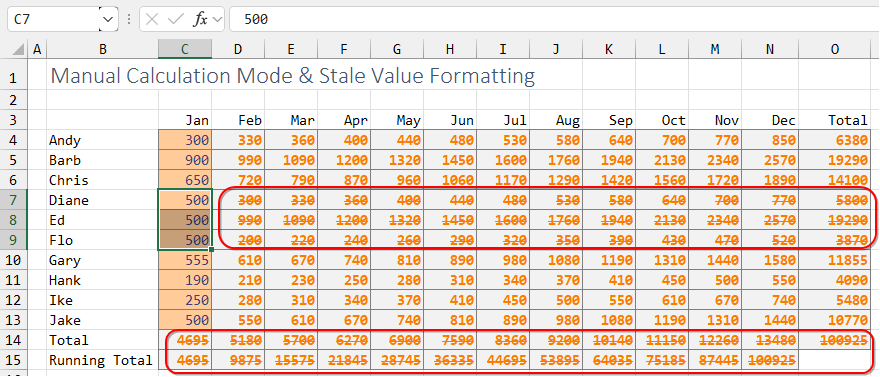If you select a cell that contains a stale value, a yellow triangle symbol appears next to the cell. Open the drop-down menu, and you have choices to Calculate Now, Switch to Automatic Calculation, Disable Format Stale Cells, or Ignore Error for this cell.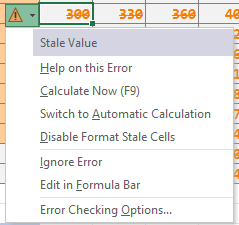### Stale Values in Automatic Calculation Mode

There can be cases where you’ll see stale values even if you’re using Automatic calculation mode. To test this, I built a worksheet with 20 RANDBETWEEN functions and 20 million formulas based on those 20 random cells. If you press F9 to generate new random numbers, the Excel status bar will show the progress of the calculation. Figure 5 shows the spreadsheet is 24% complete with the calculation.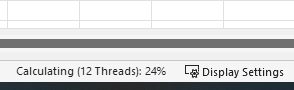If you press the Esc key, Excel will temporarily pause the calculation, and all of the uncalculated cells will show the stale value formatting, as shown in Figure 6.

After pressing the Esc key, you can prevent Excel from calculating by rapidly entering values in new cells. But as soon as you pause your data entry for a few seconds, Excel will automatically begin calculating.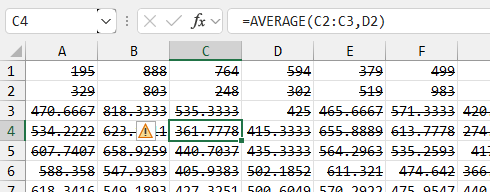### Python, Data Tables, and Partial Calculation Mode

There is a What-If Analysis drop-down on the Data tab in the Excel ribbon. As shown in Figure 7, the third choice in this drop-down menu is called Data Table, which allows you to perform sensitivity analyses.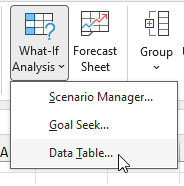In Figure 8, a simple model in C18:C21 accepts input values for Loan Amount, Term, and Interest Rate. A formula in C21 calculates the monthly payment.

To use the Data Table functionality, you’d enter various values for one input cell to the right of the monthly payment formula. These are the values shaded in blue in Figure 8. Below the monthly payment formula, enter various values for second input cells. These are shaded in green in Figure 8.

Select C21:J27 and then Formulas, What-If Analysis, Data Table. In the Data Table dialog box, you would specify that Excel should iterate through the values in the top row and place them in cell C18. The items along the left column would be placed in C19.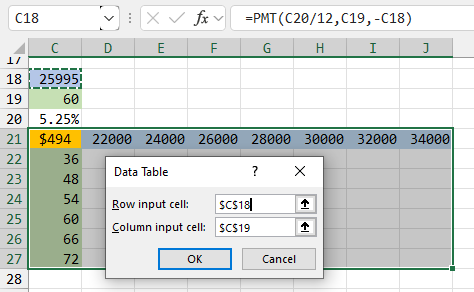When you press OK, Excel will run this simple model with each combination of the six input cells along the left and the seven input cells across the top. This means the model will be calculated 42 times.

The result, shown in Figure 9, is a Table function that generates the monthly payment for various combinations of the two input cells.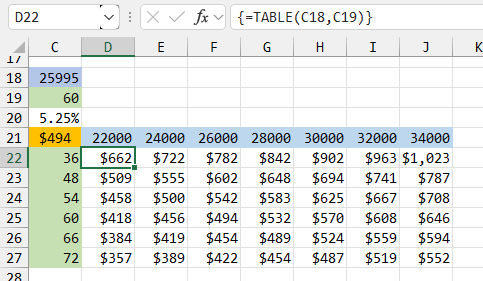Set the calculation mode to Partial. Type a new interest rate in cell C20. The formula in C21 is updated, but all of the results of the Table function are marked as stale values (see Figure 10). You can press F9 or click Calculate Now on the Formulas tab to calculate the table.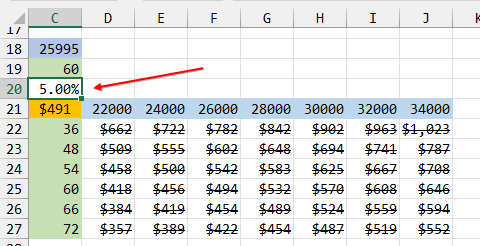Note that the simple model shown above would normally calculate in less than a second. But it’s possible to build complex models with thousands of formulas and then to build a table that runs thousands of formulas hundreds of times. The Partial calculation mode is particularly useful for these larger models.

Microsoft began rolling out a preview of Python built in to Excel in late August 2023. The Partial calculation mode will also pause calculation of any Python code in the workbook. Watch for an article about the Python preview in Excel in a future issue of Strategic Finance.

### Should These Really Be Stale?

Figure 11 shows a very simple scenario. Cell C38 has the value of 100. Select cells D38:K38. Type a formula to add 10 to the cell to the left. Fill the entire selection by pressing Ctrl+Enter.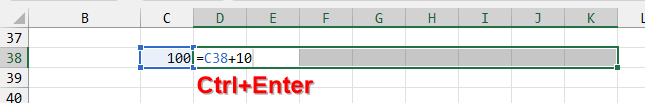In my experience, the active cell of D10 will always be correct after this sequence. In Figure 12, it’s easy to see that the values in E38:K38 are also correct. But they’re marked as stale values. Microsoft says that depending on the complexity of the worksheet, the values in E38:K38 may not always be correctly calculated at this point. To be overly cautious, Excel will mark these values as stale even though they happen to be correct in this case.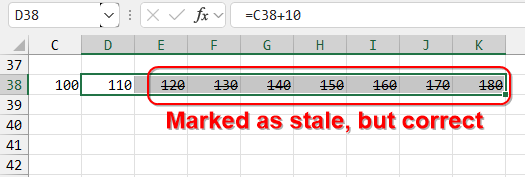### Excel Options and Calculation Mode

The calculation options from Figure 1 are also available in Excel Options, as shown in Figure 13. Note the “Partial” at the top and “Cells containing stale values” near the bottom.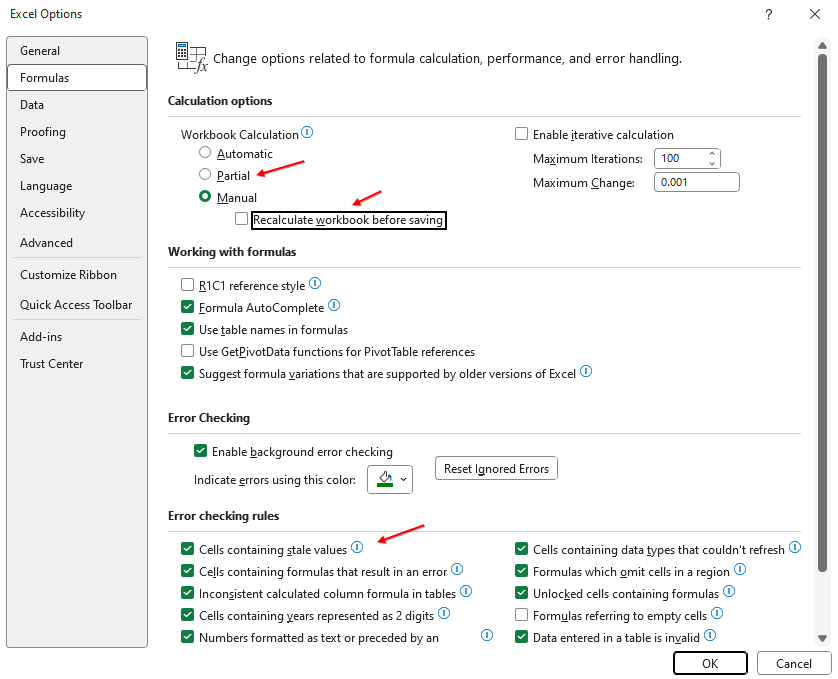Also in Figure 13, there’s an option to prevent the workbook from being recalculated before saving. This option is important for people who have workbooks that take hours to calculate.

The new stale value formatting provides an extra visual indicator that the entire workbook hasn’t been calculated.